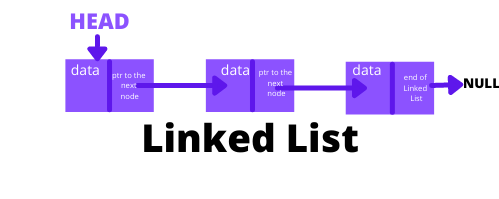# Linked Lists and it's implementation in C

Written by Richa Kiran on Jun 13th, 2021 Views

THIS IS MY FIRST BLOG EVER! and I'm gonna talk about linked list data structure in this blog.

Let's first understand some basic things about a Linked List before understanding the code.

Let's say this block is called 'Node'.This Node has two segments. The first segment stores it's own data and the second segment contains address to some other Node.Imagine a bunch of Nodes, each node containing address to some other Node. That's how a Linked List is formed.The Head Node is the Node where the Linked List starts. The last element of a Linked List points at 'NULL'. So Basically, Nodes are chunks of memory randomly distributed inside the computer's memory. That's why Nodes contain the address of the next Node so as to be a part of an ordered List. I hope this made the idea of a linked list a little clearer to you.

So now let's dig into the coding part of a Node:

Suppose we have to make a Node that stores int data, it'll be represented as -

``````struct node{
int data; //the data stored
struct node *next; //pointer to the next Node
};
``````

Now let's make a function to create a node. All this function is gonna do is allocate a piece of memory to the newly created node, it sets its value equal to whatever the value of argument variable is and the pointer to the next node is initially set to null.

``````typedef struct node{
int data;
struct node *next;
}NODE;

NODE *createNode(int data){
NODE *z = (NODE *)malloc(sizeof(NODE));//allocate some memory to the new node;
z->data = data; //assign the data to the new node's data segment
z->next = NULL; //initially set next of this node equal to null
return z;
}
``````

Next, we make a function for printing the Linked List.

``````void printll(NODE *head){
while(p!=NULL){
printf("%d=> ", p->data);
p = p->next;
}
printf("\n");
}

``````

This function basically accepts a pointer as an argument. Prints the data stored in the node that its pointing at. Then it sets itself to the next of that Node and this continues until the next of node is equal to null, which marks the end of the list.

So now that we know some basic programming for Nodes, lets get into how we can join two Nodes. The most basic way to do that is to make two nodes and set one's next equal to the second node's address. Like-

``````int main(){
NODE *a,*b;
a = createNode(1) //assigning 1 as value to the node 'a'
b = createNode(2) //assigning 2 as value to the node 'b'
//joining the two nodes;
a->next = b;
//lets make a third node;
c = createNode(3);
//link it to the next of b;
b->next = c;
}
``````

But, what if we have hundreds of such Nodes to link? This is obviously not the best way to do it.

That's why, now we'll make a function that will automatically link one Node to another.

What we're gonna do now is, first we create a new node. First it checks if the head is pointing to null, if so, it sets the new node equal to the head. Otherwise, it traverses the whole linked list till it reaches the end and then links the new node at the end of it.

``````NODE *CreateLinkedList(int data, NODE *head){
NODE *newnode = createNode(data);
}
else{
while(temp->next != NULL){
temp = temp->next;
}//temp reaches end of the linked list;
temp->next = newnode;//the node that was previously pointing to NULL
// is now pointing at the newnode;
}
}
``````

The final program looks something like this:

``````#include <stdio.h>
#include <stdlib.h>
typedef struct node{
int data;
struct node *next;
}NODE;
NODE *createNode(int data){
NODE *z = (NODE *)malloc(sizeof(NODE));
z->data = data;
z->next = NULL;
return z;
}

while(p!=NULL){
printf("%d=> ", p->data);
p = p->next;
}
printf("\n");
}

NODE *newnode = createNode(data);
}
else{
while(temp->next != NULL){
temp = temp->next;
}//temp reaches end of the linked list;
temp->next = newnode;//the node that was previously pointing to NULL
// is now pointing at the newnode;
}
}

int main(){
int choice = 1;
do{
int val;
printf("enter the value to be inserted: ");
scanf(" %d", &val);
printf("Enter 1 for continue and 0 for exit :");
scanf(" %d", &choice);
}while(choice==1);

}
``````

So, this is how we create a basic linked list. There are many other operations in a linked list like inserting element at a particular index, deleting elements and so on.

I had a hard time understanding the concept of linked lists when I first came across it. I had to do a lot of searching from random resources online to be able to understand and implement this concept, being totally new to Data Structure and Algorithms. I've tried to consolidate all the knowledge that I have of basic implementation of a linked list through this blog. Hope you like it!